# RS Aggarwal Solutions for Class 6 Maths Chapter 16 Triangles

RS Aggarwal Solutions for Class 6 Maths Chapter 16 Triangles are given here in simple PDF format. Students are advised to practice RS Aggarwal textbook questions meticulously. Practising helps the students improve their performance in the examination with high marks and thereby, boosting their confidence in Mathematics. Students who refer RS Aggarwal textbook solutions will understand the chapter effectively clearing all their doubts.

Mathematics experts solved RS Aggarwal textbook questions for Class 6 Maths Chapter 16 which is available in PDF format. Download PDF of Class 6 Chapter 16 in their respective links.

## Download PDF of RS Aggarwal Solutions for Class 6 Maths Chapter 16 Triangles.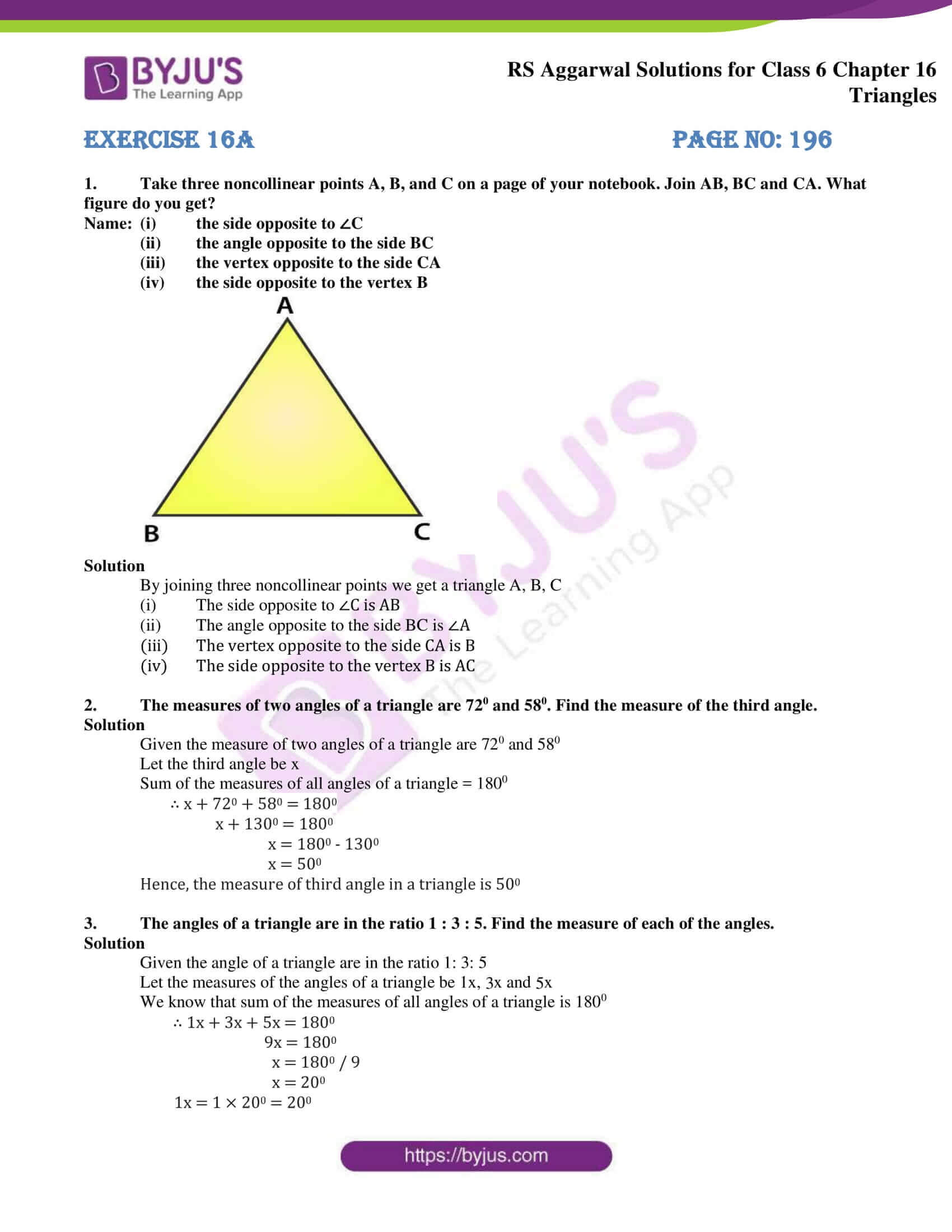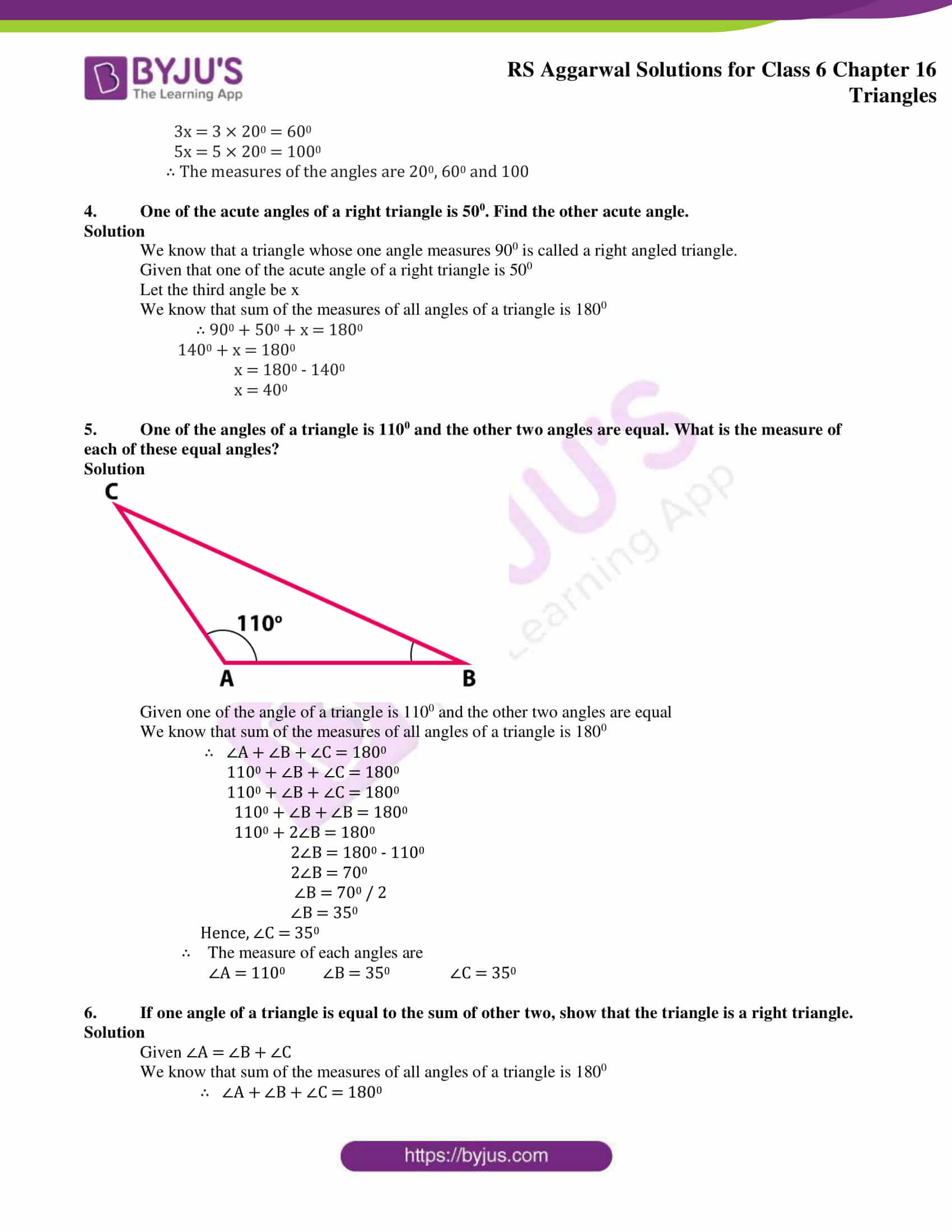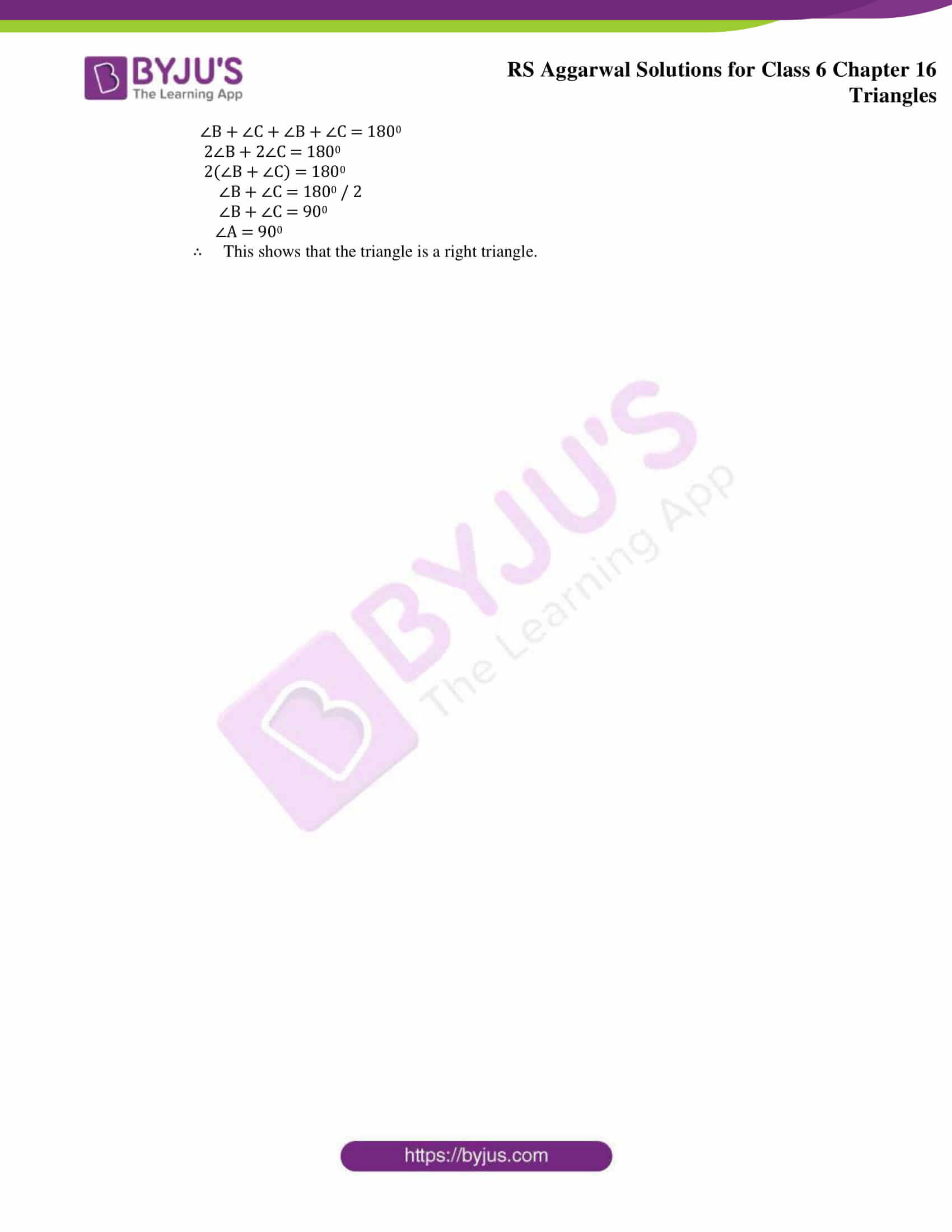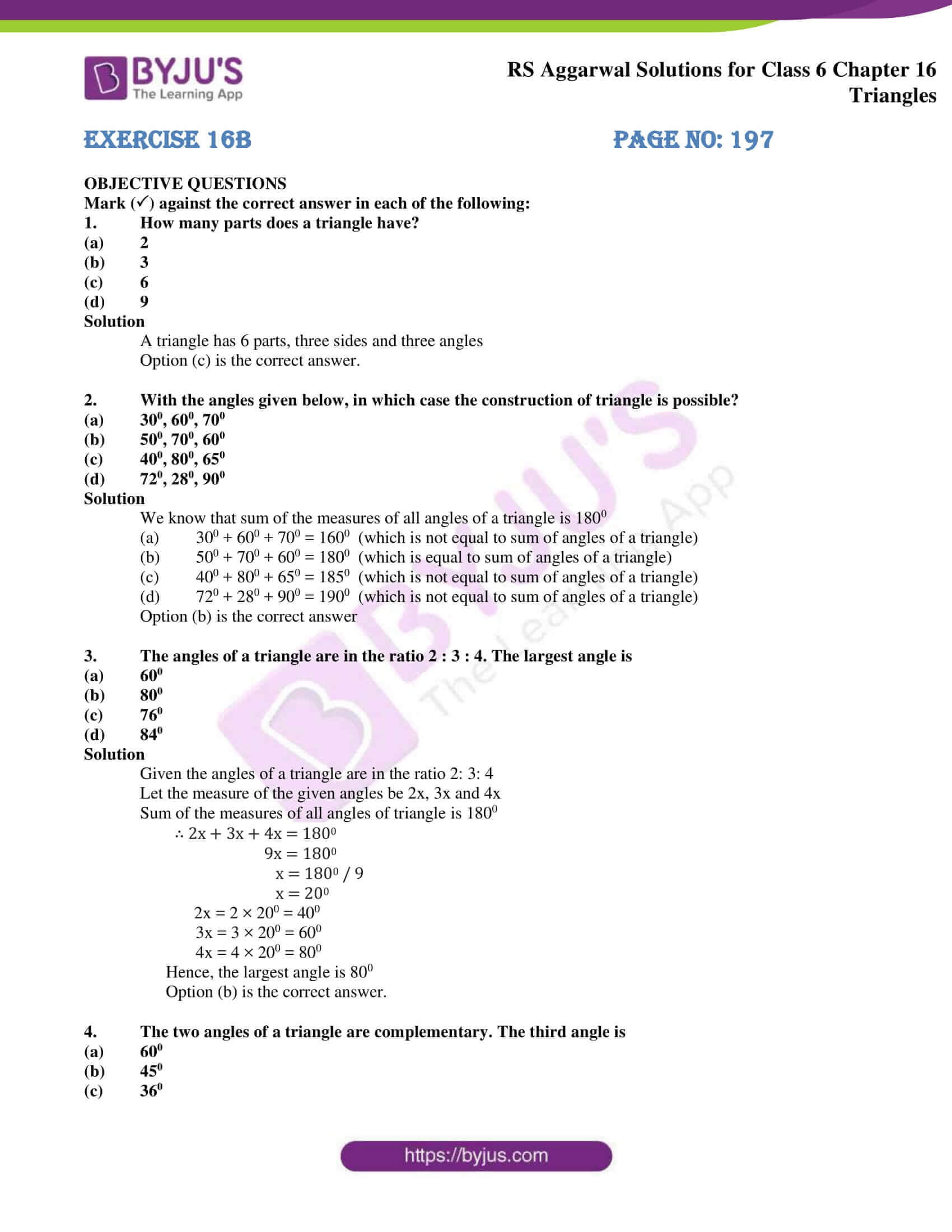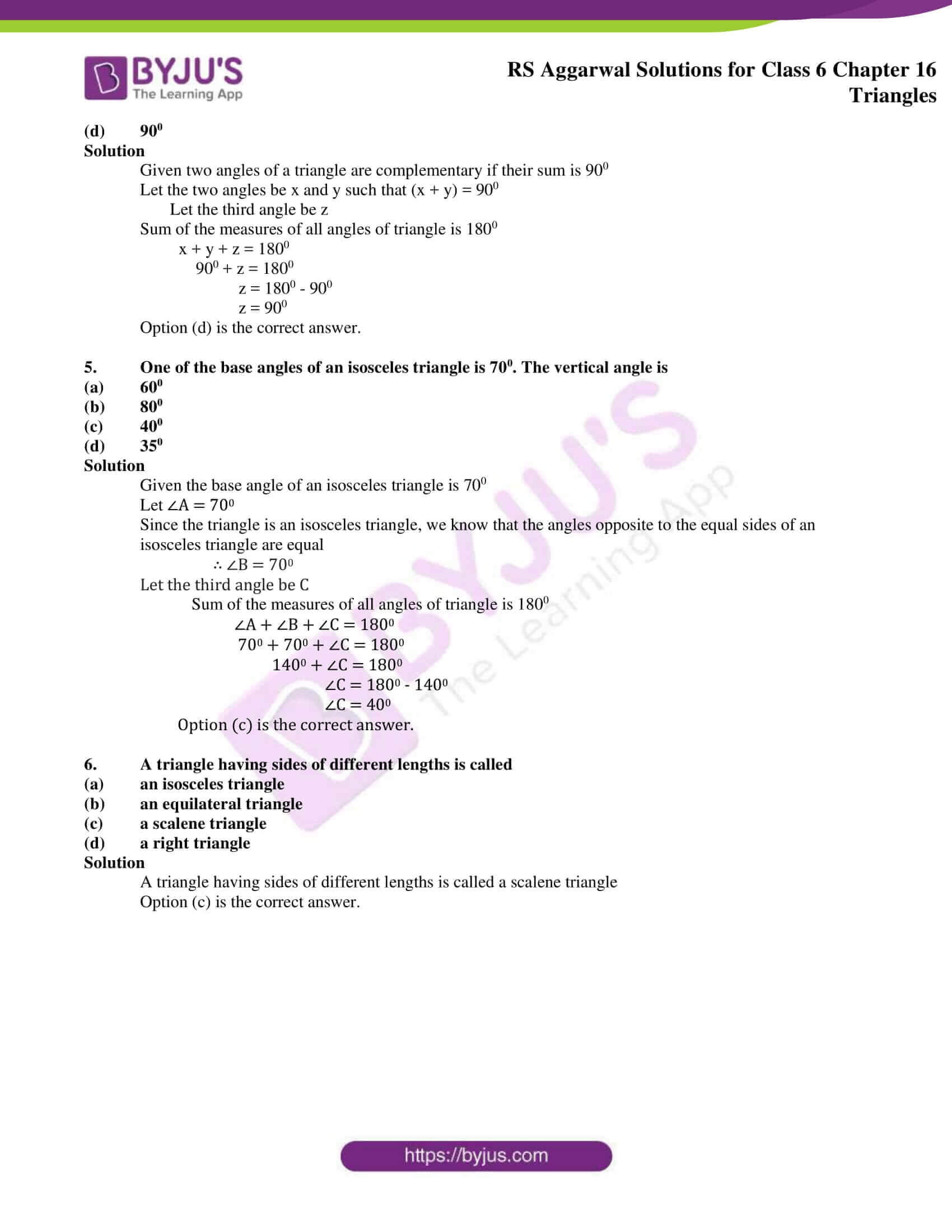### Access answers of RS Aggarwal Solutions for Class 6 Maths Chapter 16 – Triangles

Exercise 16A PAGE NO: 196

1. Take three noncollinear points A, B, and C on a page of your notebook. Join AB, BC and CA. What figure do you get?

Name: (i) the side opposite to ∠C

(ii) the angle opposite to the side BC

(iii) the vertex opposite to the side CA

(iv) the side opposite to the vertex B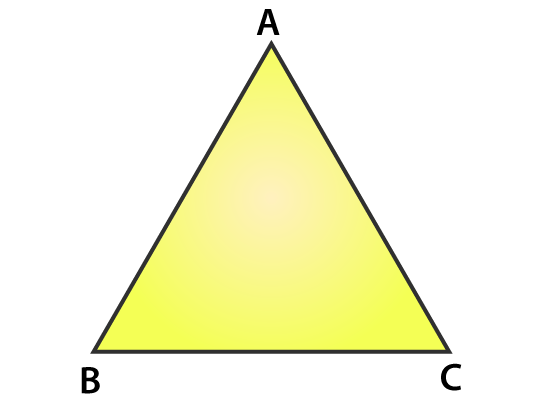Solution

By joining three noncollinear points we get a triangle A, B, C

(i) The side opposite to ∠C is AB

(ii) The angle opposite to the side BC is ∠A

(iii) The vertex opposite to the side CA is B

(iv) The side opposite to the vertex B is AC

2. The measures of two angles of a triangle are 720 and 580. Find the measure of the third angle.

Solution

Given the measure of two angles of a triangle are 720 and 580

Let the third angle be x

Sum of the measures of all angles of a triangle = 1800

∴ x + 720 + 580 = 1800

x + 1300 = 1800

x = 1800 – 1300

x = 500

Hence, the measure of third angle in a triangle is 500

3. The angles of a triangle are in the ratio 1 : 3 : 5. Find the measure of each of the angles.

Solution

Given the angle of a triangle are in the ratio 1: 3: 5

Let the measures of the angles of a triangle be 1x, 3x and 5x

We know that sum of the measures of all angles of a triangle is 1800

∴ 1x + 3x + 5x = 1800

9x = 1800

x = 1800 / 9

x = 200

1x = 1 × 200 = 200

3x = 3 × 200 = 600

5x = 5 × 200 = 1000

∴ The measures of the angles are 200, 600 and 100

4. One of the acute angles of a right triangle is 500. Find the other acute angle.

Solution

We know that a triangle whose one angle measures 900 is called a right angled triangle.

Given that one of the acute angle of a right triangle is 500

Let the third angle be x

We know that sum of the measures of all angles of a triangle is 1800

∴ 900 + 500 + x = 1800

1400 + x = 1800

x = 1800 – 1400

x = 400

5. One of the angles of a triangle is 1100 and the other two angles are equal. What is the measure of each of these equal angles?

Solution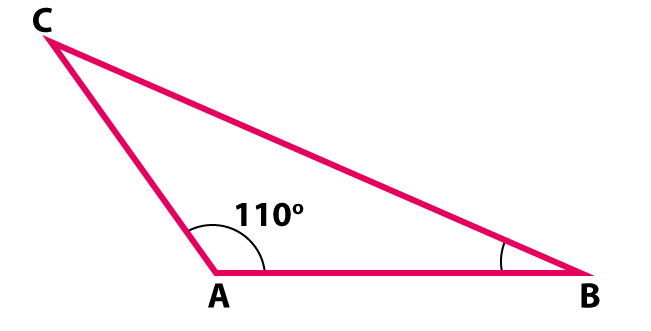Given one of the angle of a triangle is 1100 and the other two angles are equal

We know that sum of the measures of all angles of a triangle is 1800

∴ ∠A + ∠B + ∠C = 1800

1100 + ∠B + ∠C = 1800

1100 + ∠B + ∠C = 1800

1100 + ∠B + ∠B = 1800

1100 + 2∠B = 1800

2∠B = 1800 – 1100

2∠B = 700

∠B = 700 / 2

∠B = 350

Hence, ∠C = 350

∴ The measure of each angles are

∠A = 1100 ∠B = 350 ∠C = 350

6. If one angle of a triangle is equal to the sum of other two, show that the triangle is a right triangle.

Solution

Given ∠A = ∠B + ∠C

We know that sum of the measures of all angles of a triangle is 1800

∴ ∠A + ∠B + ∠C = 1800

∠B + ∠C + ∠B + ∠C = 1800

2∠B + 2∠C = 1800

2(∠B + ∠C) = 1800

∠B + ∠C = 1800 / 2

∠B + ∠C = 900

∠A = 900

∴ This shows that the triangle is a right triangle.

Exercise 16B PAGE NO: 197

OBJECTIVE QUESTIONS

Mark () against the correct answer in each of the following:

1. How many parts does a triangle have?

(a) 2

(b) 3

(c) 6

(d) 9

Solution

A triangle has 6 parts, three sides and three angles

Option (c) is the correct answer.

2. With the angles given below, in which case the construction of triangle is possible?

(a) 300, 600, 700

(b) 500, 700, 600

(c) 400, 800, 650

(d) 720, 280, 900

Solution

We know that sum of the measures of all angles of a triangle is 1800

(a) 300 + 600 + 700 = 1600 (which is not equal to sum of angles of a triangle)

(b) 500 + 700 + 600 = 1800 (which is equal to sum of angles of a triangle)

(c) 400 + 800 + 650 = 1850 (which is not equal to sum of angles of a triangle)

(d) 720 + 280 + 900 = 1900 (which is not equal to sum of angles of a triangle)

Option (b) is the correct answer

3. The angles of a triangle are in the ratio 2 : 3 : 4. The largest angle is

(a) 600

(b) 800

(c) 760

(d) 840

Solution

Given the angles of a triangle are in the ratio 2: 3: 4

Let the measure of the given angles be 2x, 3x and 4x

Sum of the measures of all angles of triangle is 1800

∴ 2x + 3x + 4x = 1800

9x = 1800

x = 1800 / 9

x = 200

2x = 2 × 200 = 400

3x = 3 × 200 = 600

4x = 4 × 200 = 800

Hence, the largest angle is 800

Option (b) is the correct answer.

4. The two angles of a triangle are complementary. The third angle is

(a) 600

(b) 450

(c) 360

(d) 900

Solution

Given two angles of a triangle are complementary if their sum is 900

Let the two angles be x and y such that (x + y) = 900

Let the third angle be z

Sum of the measures of all angles of triangle is 1800

x + y + z = 1800

900 + z = 1800

z = 1800 – 900

z = 900

Option (d) is the correct answer.

5. One of the base angles of an isosceles triangle is 700. The vertical angle is

(a) 600

(b) 800

(c) 400

(d) 350

Solution

Given the base angle of an isosceles triangle is 700

Let ∠A = 700

Since the triangle is an isosceles triangle, we know that the angles opposite to the equal sides of an isosceles triangle are equal

∴ ∠B = 700

Let the third angle be C

Sum of the measures of all angles of triangle is 1800

∠A + ∠B + ∠C = 1800

700 + 700 + ∠C = 1800

1400 + ∠C = 1800

∠C = 1800 – 1400

∠C = 400

Option (c) is the correct answer.

6. A triangle having sides of different lengths is called

(a) an isosceles triangle

(b) an equilateral triangle

(c) a scalene triangle

(d) a right triangle

Solution

A triangle having sides of different lengths is called a scalene triangle

Option (c) is the correct answer.

### RS Aggarwal Solutions for Class 6 Maths Chapter 16 Triangles

Chapter 16 – Triangles consists of 2 exercises. Each and every question of RS Aggarwal textbook has been solved thoroughly. Let’s have a glance at the topics included in Triangles.

• Congruent triangles
• Interior and exterior of a triangle
• Various types of triangles
• Naming triangles by considering their angles
• Angle sum property of a triangle

Also, access RS Aggarwal Solutions for Class 6 Maths Chapter 16 Exercises

Exercise 16A

Exercise 16B

### Chapter Brief of RS Aggarwal Solutions for Class 6 Maths Chapter 16 – Triangles

A polygon with three line segments and three vertices is known as a Triangle. Types of triangles are equilateral, isosceles, scalene, acute and obtuse triangles. Traffic signs, roof, staircase and ladder, buildings, monuments, towers etc. are examples of triangles used in daily life.